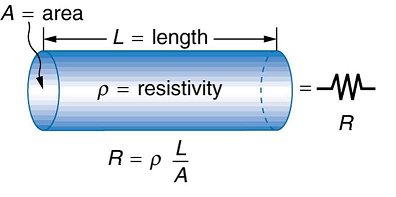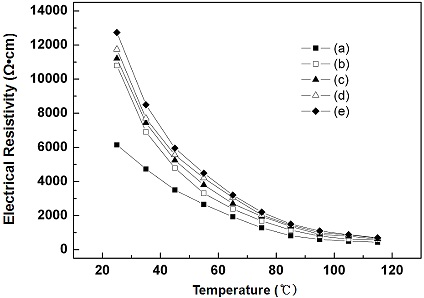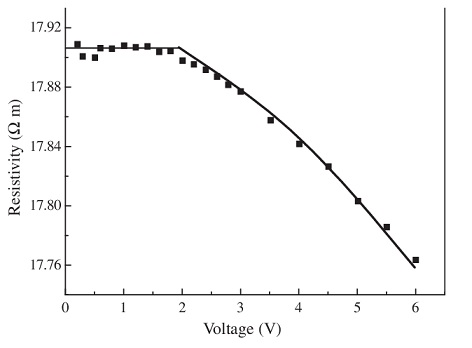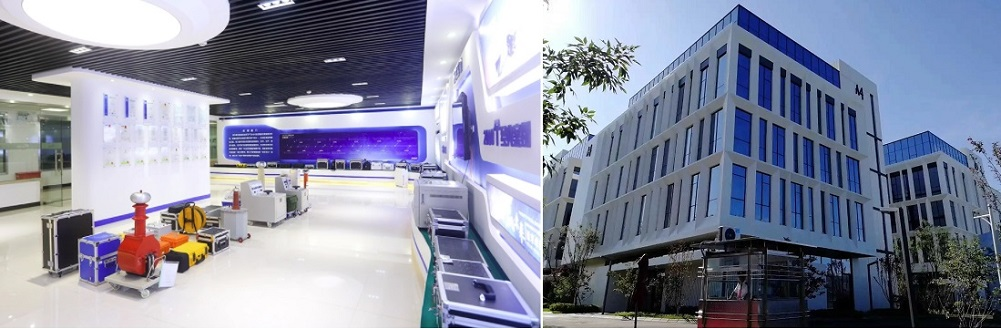Technology Literature

# What are the main factors that affect resistivity?

Resistivity is a physical quantity used to represent the resistance characteristics of various substances. The resistance of a conductor with a length of 1m and a cross-sectional area of 1m2 made of a certain material is numerically equal to the resistivity of this material. At a certain temperature, the resistance of the material is:

ρ=RA/L

where ρ is the resistivity, L is the length of the material, and A is the cross-sectional area of the material. It can be seen that the resistance of the material is proportional to the length of the material, that is, when the material and cross-sectional area are constant, the longer the length, the greater the resistance of the material; and it is inversely proportional to the cross-sectional area of the material, that is, when the material and the length are not the same. When changing, the larger the cross-sectional area, the smaller the resistanceResistivity is a physical quantity that reflects the electrical conductivity of a conductor. Small resistivity, good electrical conductivity; large resistivity, poor electrical conductivity. The size of the resistivity is determined by the properties of the material itself. In addition, the resistivity is also affected by the following factors:

a. Temperature and humidity

The resistance of general materials decreases with increasing temperature and humidity. In contrast, surface resistance (rate) is sensitive to ambient humidity, while bulk resistance (rate) is more sensitive to temperature. As humidity increases, surface leakage increases, and the body's conduction current increases. When the temperature increases, the moving speed of the carriers increases, and the absorption current and conduction current of the medium will increase accordingly. It is reported that the resistance of the general medium at 70C is only 10% of that at 20C. Therefore, to measure the resistance of a material, it is necessary to specify that the sample is in equilibrium with the ambient temperature and humidity.# Relationship between the electrical resistivity (ρ) and the temperature for (a) MnNiCoO4, (b) MnNiCo0.95Al0.05O4, (c) MnNiCo0.90Al0.10O4, (d) MnNiCo0.85Al0.15O4, and (e) MnNiCo0.80Al0.20O4 NTC thermistors.

b. Test voltage (electric field strength)

Dielectric resistance (rate) values generally do not remain constant over a wide voltage range, which does not apply Ohm's law. At room temperature, in the lower voltage range, the on-current increases linearly with the applied voltage, and the resistance value remains unchanged. After a certain voltage is exceeded, the movement of ions increases, the on-current increases faster than the test voltage, and the resistance of the material decreases rapidly. Therefore, the higher the test voltage applied, the lower the resistance of the material, resulting in possible large differences in the resistance test of materials with different voltages.# Resistivity vs. voltage for CFRC with fiber content of 0.05 vol.% under the condition of small voltage.

It is worth noting that the determinant of the resistance change of the material is the test electric field strength, not the test voltage. Under the same test voltage, the test results of resistivity will be different if the distance between different tested electrodes is different. The smaller the distance between the positive and negative electrodes, the smaller the test value.

c. Test time
The DC voltage exerts a certain pressure on the material under test, and the current value of the material under test stabilizes not instantaneously, but has a decay process. While pressurizing, the greater the charging current that flows, the longer the current is absorbed, and the more stable the final conductance is. The higher the measured resistance value, the longer it will take to reach equilibrium. Therefore, in order to correctly read the measured value of the test resistance, the pressure reading should be taken after a stable value or 1 minute.

In addition, the high insulation resistance value is also related to its charging history. In order to accurately evaluate the electrostatic properties of the material, when the material is in the resistance (rate) test, its power consumption should be treated first, and it should be left for a period of time, preferably 5 minutes, and then the measurement procedure test is carried out. Generally speaking, for the test of a material, at least 3 to 5 samples are randomly selected for testing, and the average value is taken as the test result.

d. Leak testing equipment
During the test, the insulation resistance of the circuit connection is not high, and the measured object is often improperly sampled, such as parallel sampling resistance, which may have a greater impact on the measurement results. do this:
In order to reduce the measurement error, protection technology should be used in the leakage current of the installed circuit protective conductor to basically eliminate the influence of stray current on the test results;
Due to the surface ionization of the high-voltage line, there is a certain leakage to the ground, so the high-voltage output cable should be made of high-insulation, large-diameter high-voltage lines as much as possible, and the connection should be as short as possible to reduce the tip and prevent corona discharge;
Test benches and supports for insulating materials such as polyethylene and teflon to avoid the reasons for such tests being reduced.

e. External interference

The insulating material adopts DC high voltage, and the current passing through the sample is very small, which is easily affected by external interference, resulting in a large test error. Thermoelectric potential and contact potential are generally small and can be ignored; the electrolytic potential is mainly wet samples generated by different metal contacts, only about 20mV, and static testing does require relatively low humidity. When testing in a dry environment, Electrolytic potential can be eliminated. Therefore, the external interference is mainly the coupling of stray current or potential generated by electrostatic induction.

Commonly used metal conductor resistivity ρ(Ω·m):
(1) Silver 1.65 × 10-8
(2) Copper 1.75 × 10-8
(3) Gold 2.40×10-8
(4) Aluminum 2.83 × 10-8
(5) Tungsten 5.48 × 10-8
(6) Iron 9.78 × 10-8
(7) Platinum 2.22 × 10-7
(8) Manganese Bronze 4.4 × 10-7
(9) Mercury 9.6 × 10-7
(10) Constantan 5.0 × 10-7
(11) Nickel-chromium alloy 1.0 × 10-6
(12) Iron-chromium-aluminum alloy 1.4 × 10-6
(13) Al-Ni-Fe alloy 1.6 × 10-6

It can be seen that the resistivity of pure metals in conductors is small, and the resistivity of alloys is large. The resistivity of silver is the smallest, but the price of silver is expensive. Usually, silver is rarely used as a wire, and it is only used for special needs. The wire is generally made of copper or aluminum with a lower resistivity. Aluminum is cheaper than copper, so aluminum A lot of wires are used. The resistance wires of electric furnaces and resistors are generally made of alloys with higher resistivity.

The resistivity of various materials changes with temperature, and the resistivity of metals increases with temperature, so the resistance of metal conductors also increases with temperature. This property of resistance can be used to make resistance thermometers ; If the change of conductor resistance with temperature is known, then the resistance of the conductor can be measured, and the temperature of the conductor can be known in turn.